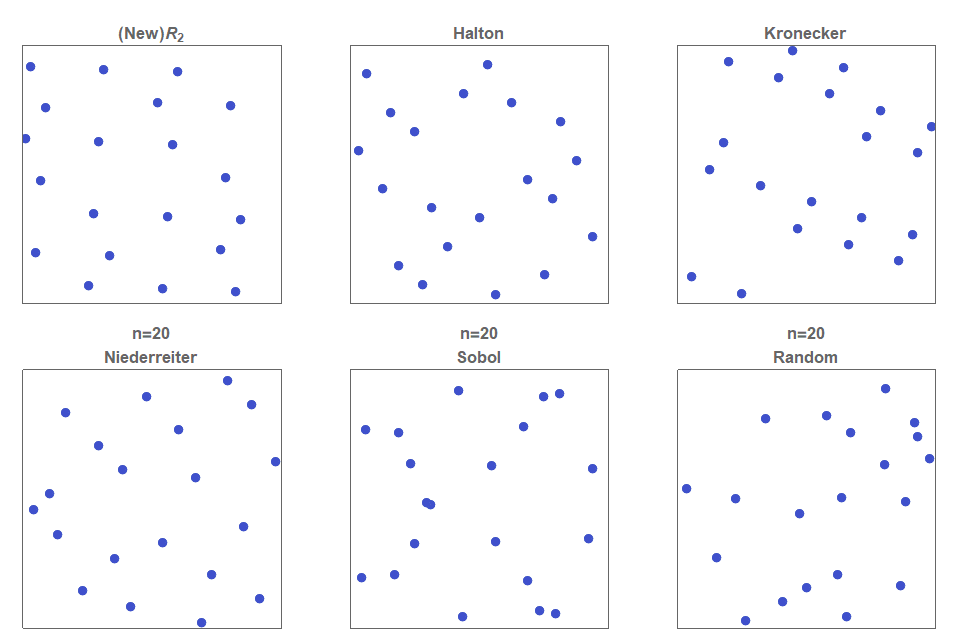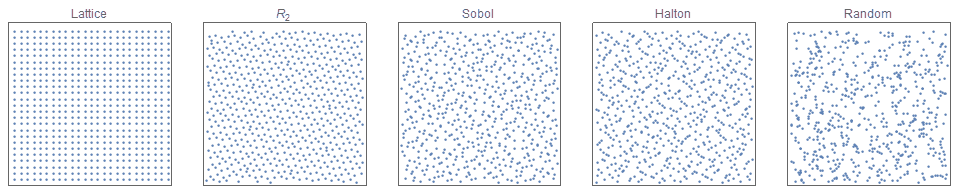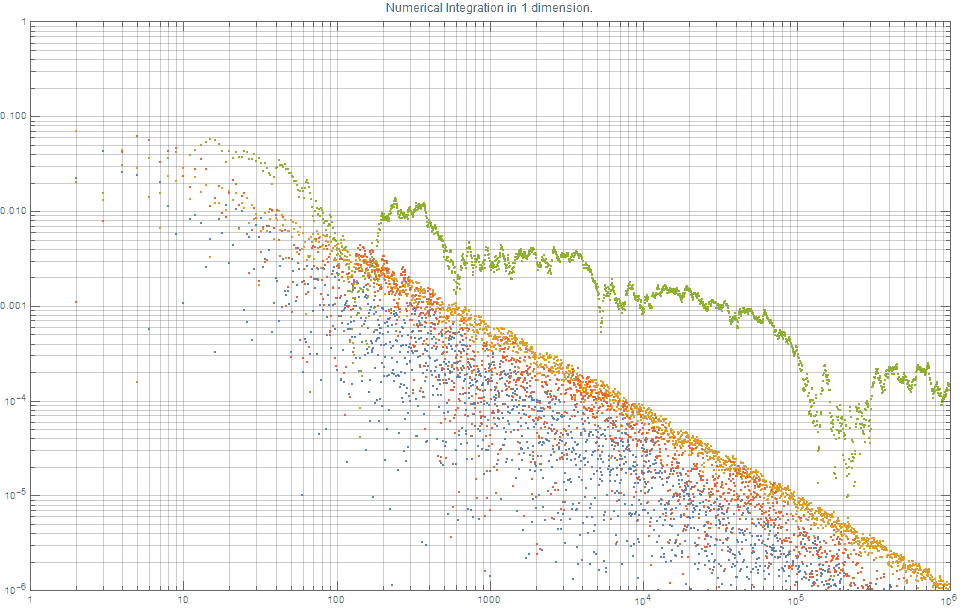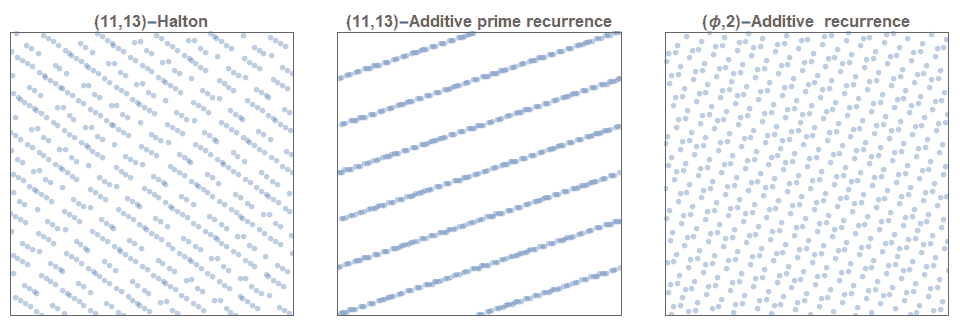# The Unreasonable Effectiveness of Quasirandom Sequences

I present a new low discrepancy quasirandom sequence that offers many substantial improvements over other popular sequences such as the Sobol and Halton sequences.Figure 1a. Comparison of the various low discrepancy quasirandom sequences. Note that the newly proposed $R$-sequence produces more evenly spaced points than any of the other methods. Furthermore, all other current methods require careful selection of basis parameters, and if not chosen carefully can lead to degeneracy (eg top right).

First published: 25th April, 2018 Last updated:    23 April, 2020

This blog post has been featured on the front page of Hacker News a few  times (Aug 2018, Oct 2020 & April 2022). See here for the awesome discussion.

Topics Covered

# Introduction: Random versus Quasirandom

In figure 1b, it can be seen that the simple uniform random sampling of a point inside a unit square exhibits clustering of points, as well as regions that contain no points at all . A low discrepancy quasirandom sequence is a fully deterministic  sequence of points that covers the entire space as uniformly as possible. They appear less regular than lattices but look more regular than random sampling (see figure 1b).

Well known quasirandom sequences include the Halton and Sobol sequences. They play a large role in many numerical computing applications, including physics, finance and in more recent decades computer graphics.

Simple random sampling is often called ‘white noise’, and low discrepancy quasirandom sequences are often called  ‘blue noise’ samples’ (although technically blue noise and low discrepancy are two similar but distinct concepts).Figure 1b. Comparison of a regular lattice (left) with 3 different quasirandom functions (middle), and a simple random distribution (right). Notice that the quasirandom distributions appear less regular than a lattice but do not have as many ‘clumps’ or ‘gaps’ as the random distribution.

# Quasirandom Sequences in 1-dimension

The methods for creating low discrepancy quasirandom sequences in one dimension are extremely well studied.

The fundamental advantage of open sequences (that is, extensible in $n$) is that if the resultant error based on a given (finite) number of terms is still too large, the sequence can be arbitrarily extended without discarding all the previously calculated points.

There are many methods to construct open sequences. One way to categorise the different types is by the method of constructing their basis (hyper)-parameters:

• irrational fractions: Kronecker, Richtmyer, Ramshaw, Weyl
• (co)prime numbers: Van der Corput, Halton, Faure
• Irreducible Polynomials : Niederreiter
• Primitive polynomials: Sobol’

This post describes a new additive recurrence $R$-sequence that falls in the first category of those sequences that are based on specially chosen irrational numbers. We compare its properties with the Halton sequence and the Sobol sequence.

This special recurrence sequence is defined as: $$R_1(\alpha): \;\; t_n = \{s_0 + n \alpha\}, \quad n=1,2,3,…$$ where $\alpha$ is any irrational number. Note that the notation $\{x\}$ indicates the fractional part of $x$. In computing, this function is more commonly expressed in the following way $$R_1(\alpha): \;\; t_n = s_0 + n \alpha \; (\textrm{mod} \; 1); \quad n=1,2,3,…$$ For $s_0 = 0$, the first few terms of the sequence, $R(\phi)$ are: $$t_n = 0.618, \; 0.236, \; 0.854, \; 0.472, \; 0.090, \; 0.708, \; 0.327, \; 0.944, \; 0.562, \; 0.180,\; 798,\; 416, \; 0.034, \; 0.652, \; 0.271, \; 0.888,…$$

It is important to note that the value of $s_0$ does not affect the overall characteristics of the sequence, and in nearly all cases is set to zero. However, especially in computing the option of $s \neq 0$ offers an additional degree of freedom that is often useful. Applying a non-zero value of $s$ is often called a toroidal shift, or a Cranley-Patterson transformation, and the resulting sequence is usually called a ‘shifted lattice sequence’.

The value of $\alpha$ that gives the lowest possible discrepancy is achieved if  $\alpha = 1/\phi$, where $\phi$ is the golden ratio. That is, $$\phi \equiv \frac{\sqrt{5}+1}{2} \simeq 1.61803398875… ;$$ It is interesting to note that there are an infinite number of other values of  $\alpha$ that also achieve optimal discrepancy, and they are all related via the Moebius transformation $$\alpha’ = \frac{p\alpha+q}{r\alpha+s} \quad \textrm{for all integers} \; p,q,r,s \quad \textrm{such that} |ps-qr|=1$$ We now compare this recurrence method to the well known van der Corput reverse-radix sequences [van der Corput, 1935] . The van der Corput sequences are actually a family of sequences, each defined by a unique hyper-parameter, b. The first few terms of the sequence for b=2 are: $$t_n^{} = \frac{1}{2}, \frac{1}{4},\frac{3}{4}, \frac{1}{8}, \frac{5}{8}, \frac{3}{8}, \frac{7}{8}, \frac{1}{16},\frac{9}{16},\frac{5}{16},\frac{13}{16}, \frac{3}{16}, \frac{11}{16}, \frac{7}{16}, \frac{15}{16},…$$ The following section compares the general characteristics and effectiveness of each of these sequences. Consider the task of evaluating the definite integral $$A = \int_0^1 f(x) \textrm{d}x$$ We may approximate this by: $$A \simeq A_n = \frac{1}{n} \sum_{i=1}^{n} f(x_i), \quad x_i \in [0,1]$$

• If the $\{x_i\}$ are equal to  $i/n$, this is the rectangle rule;
• If the $\{x_i\}$ are chosen randomly, this is the Monte Carlo method; and
• If the $\{x_i\}$ are elements of a low discrepancy sequence, this is the quasi-Monte Carlo method.

The following graph shows the typical error curves $s_n = |A-A_n|$ for approximating a definite integral associated with the function, $f(x) = \textrm{exp}(\frac{-x^2}{2}), \; x \in [0,1]$ with: (i) quasi-random points based on the additive recurrence, where  $\alpha = 1/\phi$, (blue); (ii) quasi-random points based on the van der Corput sequence, (orange); (iii) randomly selected points, (green); (iv) Sobol sequence (red).  It shows that for $n=10^6$ points, the random sampling approach results in an error of $\simeq 10^{-4}$, the van der Corput sequence results in an error of $\simeq 10^{-6}$, whilst the $R(\phi)$-sequence results in an error of $\simeq 10^{-7}$, which is $\sim$10x better than the van der Corput error and $\sim$ 1000x better than (uniform) random sampling.Figure 2. Comparison of 1-dimensional Numerical integration using various Quasirandom Monte Carlo methods. Note smaller is better. The new $R_1$ sequence (blue) and the Sobol (red) are clearly the best.

Several things from this figure are worth noting:

• it is consistent with the knowledge that the errors based on uniform random sampling asymptotically decrease by $1/\sqrt{n}$, whereas error curves based on both quasi-random sequences tend to  $1/n$.
• The results for the $R_1(\phi)$-sequence  (blue) and Sobol (red) are the best.
• It shows that the van der Corput sequence produces error rates that are far better than random, but consistently not quite as good as Sobol or the new $R_1$ sequence.

The new $R_1$ sequence, which is a Kronecker sequence using the Golden ratio,  is one of the best choices for one-dimensional Quasirandom Monte Carlo (QMC) integration methods

It should also be noted that although $\alpha = \phi$ theoretically offers the provably optimal option, $\sqrt{2}$ and is very close to optimal, and almost any other irrational value of $\alpha$ provides excellent error curves for one-dimensional integration. This is why, $\alpha = \sqrt{p}$ for any prime is very commonly used.  Furthermore, from a computing perspective, selecting a random value in the interval  $\alpha \in [0,1]$ is with almost certainty going to be (within machine precision) an irrational number, and therefore a solid choice for a low discrepancy sequence. For visual clarity, the above figure does not show the results the Niederreiter sequence as the results  are virtually indistinguishable to that of the the Sobol and $R$ sequences.  The Neiderreiter and Sobol sequences (along with their optimized parameter selection) that were used in this post were calculated via Mathematica using what is documented as “closed, proprietary and fully optimized generators provided in Intel’s MKL library”.

# Quasirandom sequences in two dimensions

Most current methods to construct higher dimension low discrepancy simply combine (in a component-wise manner), $d$ one-dimensional sequences together. For brevity, this post mainly focuses on describing the Halton sequence [Halton, 1960] , Sobol sequence and the $d-$dimensional Kronecker sequence.

The Halton sequence is constructed simply by using $d$ different one-dimensional van de Corput sequence each with a base that is relatively prime to all the others. That is, pairwise co-prime. By far, the most frequent selection, due to its obvious simplicity and sensibility, is to select the first $d$ primes. The distribution of the first 625 points defined by the (2,3)-Halton sequence is show in figure 1. Although many two-dimensional Halton sequences are excellent sources for low discrepancy sequences, it is also well known that many are highly problematic and do not exhibit low discrepancies. For example, figure 3 shows that the (11,13)-Halton sequence produces highly visible lines. Much effort has gone into methods of selecting which pairs of ( $p_1, p_2)$ are exemplars and which ones are problematic. This issue is even more problematic in higher dimensions.

Kronecker recurrence methods generally suffer even greater challenges when generalizing to higher dimensions. That is, although using $\alpha = \sqrt{p}$ produces excellent one-dimensional sequences, it is very challenging to even find pairs of prime numbers  to be used as the basis for the two dimensional case that are not problematic! As a way around this, some have suggested using other well-known irrational numbers, such as the $\phi,\pi,e, …$. These produce moderately acceptable solutions but not are generally not used as they are usually not as good as a well-chosen Halton sequence. A great deal of effort has focused on these issues of degeneracy.

Proposed solutions include skipping/burning, leaping/thinning. And for finite sequences scrambling is another technique that is frequently used to overcome this problem. Scrambling can not be used to create an open (infinite) low discrepancy sequence.Figure 3. The (11,13)-Halton sequence is clearly not a low discrepancy sequence (left). Nor is the (11,13)-prime based additive recurrence sequence (middle). Some 2-dimensional additive recurrence sequences that incorporate well known irrational numbers are reasonably good (right).

Similarly, despite the generally better performance of the Sobol sequence, its complexity and more importantly the requirement of very careful choices of its hyperparameters makes it not as inviting.

• Thus reiterating, in $d$-dimensions:
• the typical Kronecker sequences require the selection of $d$ linearly independent irrational numbers;
• the Halton sequence requires $d$ pairwise coprime integers; and
• the Sobol sequence requires selecting $d$ direction numbers.

The new $R_d$ sequence is the only $d$-dimensional low discrepancy quasirandom sequence that does not require any selection of basis parameters.

#

## Generalizing the Golden Ratio

tl;dr In this section, I show how to construct a  new class of $d-$dimensional open (infinite) low discrepancy sequence that do not require choosing any basis parameters, and which has outstanding low discrepancy properties.

There are many possible ways to generalize the Fibonacci sequence and/or the Golden ratio. The following proposed method of generalizing the golden ratio is not new [Krcadinac, 2005]. Also the characteristic polynomial is related to many fields of algebra, including Perron Numbers, and beautiful properties (see also here). This value was conjectured to most likely be the optimal value for a related two-dimensional problem [Hensley, 2002].

For $d=3$, $\phi_3 = 1.22074408460575947536…$,

For $d>3$, although the roots of this equation do not have a closed algebraic form, we can easily obtain a numerical approximation either through standard means such as Newton-Rhapson, or by noting that for the following sequence, $R_d(\phi_d)$: $$t_0=t_1 = … = t_{d} = 1;$$ $$t_{n+d+1} \;=\;t_{n+1}+t_n, \quad \textrm{for} \; n=1,2,3,..$$

This special sequence of  constants, $\phi_d$ were called ‘Harmonious numbers‘ by architect and monk, Hans van de Laan in 1928. These special values can be expressed very elegantly as follows:

$$\phi_1 = \sqrt{1+\sqrt{1+\sqrt{1+\sqrt{1+\sqrt{1+…}}}}}$$

$$\phi_2 = \sqrt{1+\sqrt{1+\sqrt{1+\sqrt{1+\sqrt{1+…}}}}}$$

$$\phi_3 = \sqrt{1+\sqrt{1+\sqrt{1+\sqrt{1+\sqrt{1+…}}}}}$$

We also have the following very elegant property: $$\phi_d =\lim_{n\to \infty} \;\;\frac{t_{n+1}}{t_n} .$$ This sequence, sometimes called the generalized or delayed Fibonacci sequence, has been studied quite widely, [Kak 2004, Wilson 1993] and the sequence for $d=2$ is often called the Padovan sequence [Stewart, 1996, OEIS A000931], whilst the $d=3$ sequence is listed in [OEIS A079398]. As mentioned before, the key contribution of this post is to describe the explicit connection between this generalized sequence and the construction of $d-$dimensional low-discrepancy sequences.

Major Result: The following parameter-free $d-$dimensional open (infinite) sequence $R_d(\phi_d)$, has excellent low discrepancy characteristics when compared to other existing methods. $$\mathbf{t}_n = \{n \pmb{\alpha} \}, \quad n=1,2,3,…$$ $$\textrm{where} \quad \pmb{\alpha} =(\frac{1}{\phi_d}, \frac{1}{\phi_d^2},\frac{1}{\phi_d^3},…\frac{1}{\phi_d^d}),$$ $$\textrm{and} \; \phi_d\ \textrm{is the unique positive root of } x^{d+1}=x+1.$$

For two dimensions, this generalized sequence for $n=150$, is shown in figure 1. The points are clearly more evenly distributed  for the $R_2$-sequence compared to the (2, 3)-Halton sequence, the Kronecker sequence based on $(\sqrt{3},\sqrt{7})$, the Niederreiter and Sobol sequences. (Due to the complexity of the Niederreiter and Sobol sequences they were calculated via Mathematica using proprietary code supplied by Intel.) This type of sequence, where the basis vector $\pmb{\alpha}$ is a function of a single real value, is often called a Korobov sequence [Korobov 1959]

See figure 1 again for a comparison between various 2-dimensional low discrepancy quasirandom sequences.

## Code and Demonstrations

In summary in 1 dimension, the pseudo-code for the $n$-th term ($n$ = 1,2,3,….) is  defined as

g = 1.6180339887498948482
a1 = 1.0/g
x[n] = (0.5+a1*n)


In 2 dimensions, the pseudo-code for the $x$ and $y$ coordinates of the $n$-th term ($n$ = 1,2,3,….) are defined as

g = 1.32471795724474602596
a1 = 1.0/g
a2 = 1.0/(g*g)
x[n] = (0.5+a1*n)
y[n] = (0.5+a2*n)


The pseudo-code for 3 dimensions, $x$, $y$ and $z$ coordinates of the $n$-th term ($n$ = 1,2,3,….) are defined as

g = 1.22074408460575947536
a1 = 1.0/g
a2 = 1.0/(g*g)
a3 = 1.0/(g*g*g)
x[n] = (0.5+a1*n)
y[n] = (0.5+a2*n)
z[n] = (0.5+a3*n)


Template python code. (Note that Python arrays and loops start at zero!)


import numpy as np

# Using the above nested radical formula for g=phi_d
# or you could just hard-code it.
# phi(1) = 1.6180339887498948482
# phi(2) = 1.32471795724474602596
def phi(d):
x=2.0000
for i in range(10):
x = pow(1+x,1/(d+1))
return x


# Number of dimensions.
d=2

# number of required points
n=50

g = phi(d)
alpha = np.zeros(d)
for j in range(d):
alpha[j] = pow(1/g,j+1)
z = np.zeros((n, d))

# This number can be any real number.
# Common default setting is typically seed=0
# But seed = 0.5 is generally better.
for i in range(n):
z[i] = (seed + alpha*(i+1))
print(z)


I have written the code like this to be consistent with the maths conventions used throughout this post. However, for reasons of programming convention and/or efficiency there are some modifications worth considering. Firstly, as $R_2$ is an additive recurrence sequence, an alternative formulation of $z$ which does not require floating point multiplication and maintains higher levels of accuracy for very large $n$ is

 z[i+1] = (z[i]+alpha)
Secondly, for those languages that allow vectorization, the fractional function code could be vectorised as follows:
for i in range(n):
z[i] = seed + alpha*(i+1)
z = z